# Place Value

Place Value tells us what the digits of the numerals that we write down mean, based on their position in the numeral.This is Blublubox, a friendly alien from the planet BleBeep who is visiting the Earth. Blublubox is interested in hearing about our number system. His planet uses a number system with only six digits because his people only have six fingers. He's curious to know what we do, because he has noticed that we have ten fingers. He wonders if we might have ten digits in our number system.

Blublubox is quite right. Our numbers are made up of the ten digits:

0, 1, 2, 3, 4, 5, 6, 7, 8, 9
Our number system is based on a system developed by the Hindus and Arabs during the sixth or seventh century. The positions of the ten digits within a number determine their values. This system is called the Hindu-Arabic number system.

The first column of digits in our numerals is called the ones column. It can have any of the values from $0$ up to $9$, depending on the digit that is written: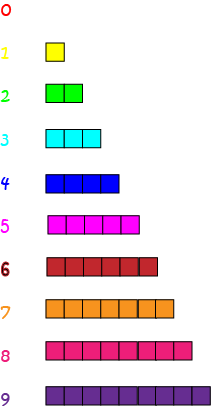The ones column can be used to write any of the values from $0$ up to $9$.

## Numbers equal to ten or more

To write numbers that have the value of ten or more, we need to introduce a second column. This column is called the tens column. Our numbers will now have two columns: a tens column, which tells us how many tens there are in our number, and a ones column, which tells us how many ones (or units) there are. The tens column is written to the left of the ones column.

We can use vertical lines of squares to stand for the number of tens, like this: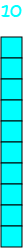Here's how we would write down the number, fourteenThe place value notation says that $14$ is made up of one ten and four ones. We can write this in expanded form as $1 \times 10 + 4 \times 1$.

For example, this is how we would write down the number, forty-six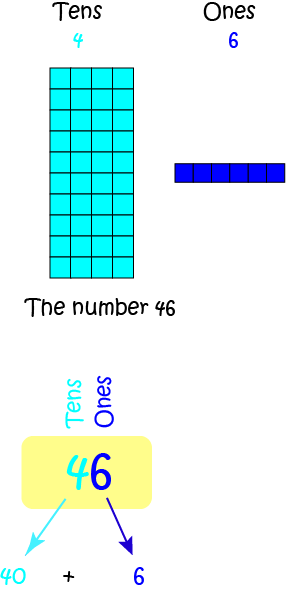The place value notation says that $46$ is made up of four tens and six ones. We can write this in expanded form as $4 \times 10 + 6 \times 1$.

## Numbers Equal to One Hundred, or More Than One Hundred

If we need to write down a numeral that is greater than or equal to one hundred, we need to introduce a new column. We call this the hundreds column. Now our numerals will have three digits that tell us the numbers of hundreds, tens and ones. We can use a square divided into one hundred squares to represent one hundred in graphical form like this: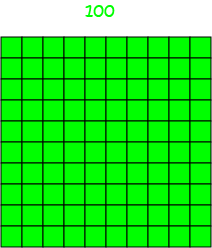For example, if we wanted to write down the numeral for one hundred and thirty-five, we could use one of the following formats:The place value notation says that $135$ is made up of one hundred, three tens and five ones. We can write this in expanded form as $1 \times 100 + 3 \times 10 + 5 \times 1$.

We can also write down the place values of each digit in $135$ as follows:

• The place value of the "1" is 100.
• The place value of the "3" is 30.
• The place value of the "5" is 5.

Let's look at another example:

The number $462$ can be written in expanded form as $4 \times 100 + 6 \times 10 + 2 \times 1$ as it includes 4 hundreds, 6 tens and 2 ones.

We can write down the place values of each digit in $462$ as follows:

• The place value of the "4" is 400.
• The place value of the "6" is 60.
• The place value of the "2" is 2.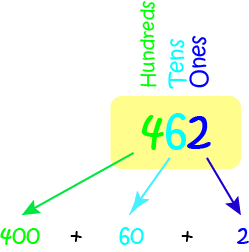## Using Zero as a Place-Holder

If a numeral has no ones, for example, we use $0$ to hold the place open so that we know what the other columns mean. For example, if we have $2$ tens, but no ones, we have the number twenty. This is how we write the numeral for twenty:The place value notation says that $20$ is made up of two tens and zero ones. We can write this in expanded form as $2 \times 10 + 0 \times 1$.

If a numeral has hundreds, but no tens, for example, we use $0$ to hold the tens place open so that we know what the hundreds and units columns mean. For example, $108$ stands for the number with one hundred, zero tens and eight ones: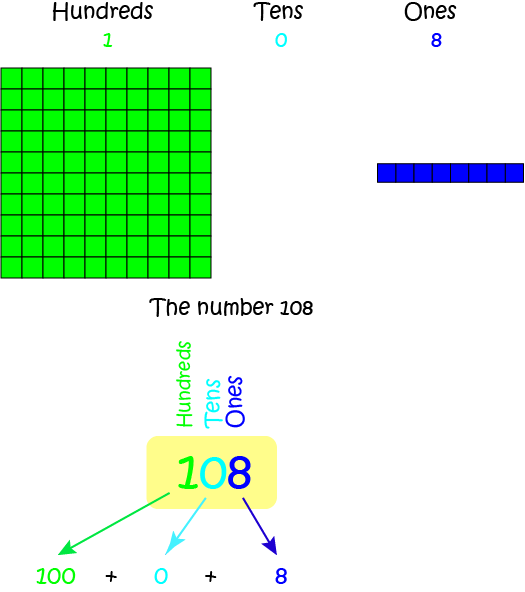## Writing Larger Numbers

If we want to write numbers in the thousands, ten thousands, hundred thousands or even millions, we need to add more columns to the left. Each new column to the left has a place value that is ten times bigger than the column immediately to its right. The position we place a digit in tells us how much that digit is worth. Here are the columns we might use to write down the number one million, three hundred and fifty-six thousand, four hundred and twenty-three:

millions hundred-thousands ten-thousands thousands hundreds tens ones
$1$ $3$ $5$ $6$ $4$ $2$ $3$
This gives us the number, $1,356,423$. That's a pretty large number, isn't it?

## Summary

The number system we use here on Earth is the Hindu-Arabic number system, based on the ten digits $0,1,2,3,4,5,6,7,8,9$, Blublubox.

There are ten digits because we have ten fingers.

The position of a digit in a number tells you its value. We call this its place value.

We use zero as a place-holder if a number has no digit of a particular value so that we know what the values of the other digits are.

It would be interesting to find out about the number system they use on the Planet Blebeep, wouldn't it? Perhaps Blublubox will tell us one day.

### Description

This mini book covers the core of Math for Foundation, Grade 1 and Grade 2 mathematics including

1. Numbers
3. Subtraction
4. Division
5. Algebra
6. Geometry
7. Data
8. Estimation
9. Probability/Chance
10. Measurement
11. Time
12. Money
13. and much more

This material is provided free of cost for Parent looking for some tricks for their Prekinder, Kinder, Prep, Year 1 and Year 2 children

### Learning Objectives

These lessons are for kids aged 4-8 with the core objective to expose their brains to concepts of addition, subtraction, division, algebra and much more.

Author: Subject Coach
You must be logged in as Student to ask a Question.